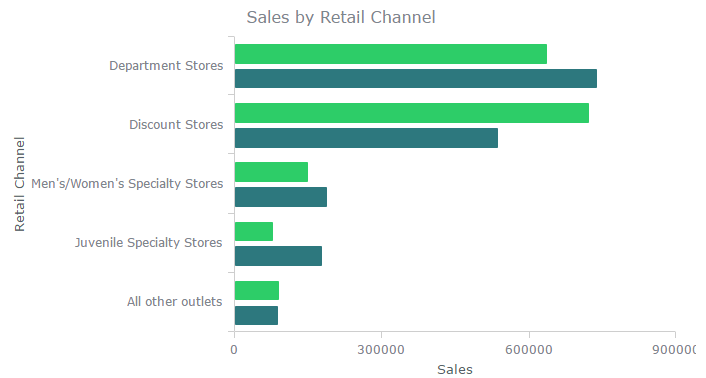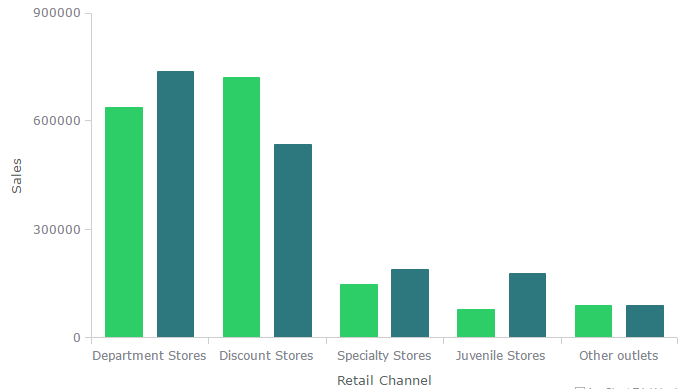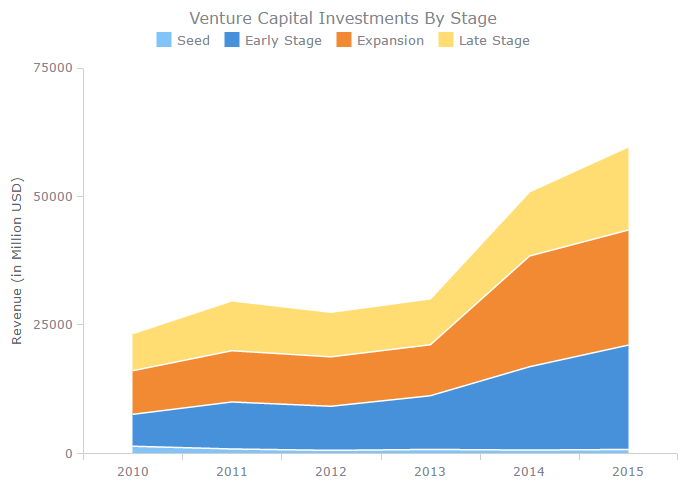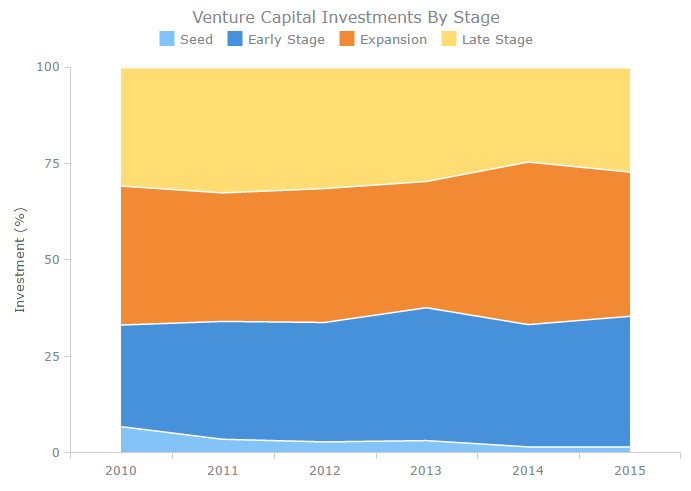# When to Use Bar Chart, Column Chart, and Area Chart

### Bar Chart and Column Chart

A Bar Chart or Bar Graph can be only used to compare values. It presents grouped data using rectangular bars whose lengths are proportional to the values that they represent. The bars can be plotted vertically or horizontally. A vertical bar chart is sometimes called a Column Chart. Bar charts are usually scaled so that all the data can fit on the chart. Bars on the chart can be arranged in any order. The point values can be compared between themselves (single-series chart) or values inside the category (multi-series chart). In case of several series points are grouped by categories.

While talking about charts, we will generally refer to different axis as dimensions and measures.The same chart can be presented as a column chart as shown below:### Area Chart

An area chart or area graph is used to display quantitative data in a graphical manner. Common uses are the comparison of two or more quantities. They can be used to represent cumulated totals using numbers or percentages over time. Use the area chart to show trends over time among related attributes. When multiple attributes are included, the first attribute is plotted as a line with color fill followed by the second attribute, and so on. Technically this chart type is based on Line Chart and represents filled area between zeroline and the line that connects data points. The same as for Line Chart, timeline scale is the most common case for the X-axis of the Area Chart, but sometimes ordinal scale can be also used.

The following area chart shows venture capital investment by stage.### 100% Stack Area Chart

100 Percent Stacked Area Charts display the data that can be put in a table on a worksheet (the items should have two coordinates or parameters). A stacked chart means that all values of one category form a whole; when we’ve got a 100% stacked chart, it means that this chart displays the comparison of the percentage value each part of the category brings to the category. Real values are not displayed, chart shows only the percentage.

This 100% stacked area chart shows the venture capital investment by stage.Get our Data Science for Finance Bundle for just $29$51.